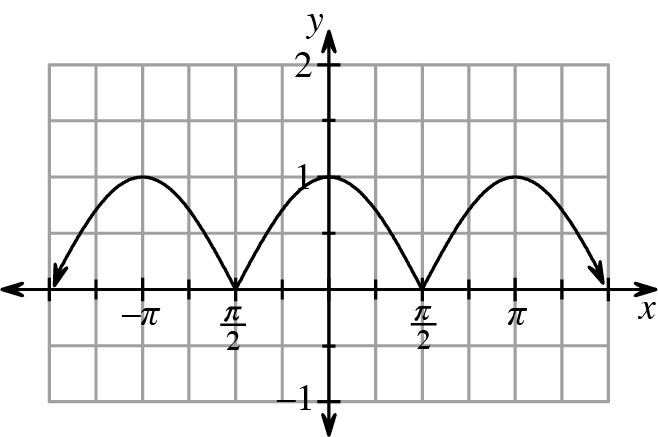### Home > CCA2 > Chapter Ch12 > Lesson 12.1.1 > Problem12-17

12-17.

Using a graph of $f(x) =$ cos($x$) for reference, graph $f(x) =|$cos($x$)$|$

How does the absolute value change a value?
How would this change look on a graph?